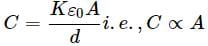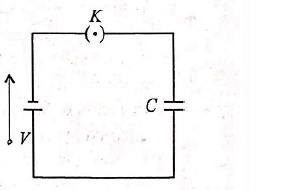NEET  >  Test: The Parallel Plate Capacitor (NCERT)

# Test: The Parallel Plate Capacitor (NCERT)

Test Description

## 5 Questions MCQ Test NCERTs at Fingertips: Textbooks, Tests & Solutions | Test: The Parallel Plate Capacitor (NCERT)

Test: The Parallel Plate Capacitor (NCERT) for NEET 2022 is part of NCERTs at Fingertips: Textbooks, Tests & Solutions preparation. The Test: The Parallel Plate Capacitor (NCERT) questions and answers have been prepared according to the NEET exam syllabus.The Test: The Parallel Plate Capacitor (NCERT) MCQs are made for NEET 2022 Exam. Find important definitions, questions, notes, meanings, examples, exercises, MCQs and online tests for Test: The Parallel Plate Capacitor (NCERT) below.
Solutions of Test: The Parallel Plate Capacitor (NCERT) questions in English are available as part of our NCERTs at Fingertips: Textbooks, Tests & Solutions for NEET & Test: The Parallel Plate Capacitor (NCERT) solutions in Hindi for NCERTs at Fingertips: Textbooks, Tests & Solutions course. Download more important topics, notes, lectures and mock test series for NEET Exam by signing up for free. Attempt Test: The Parallel Plate Capacitor (NCERT) | 5 questions in 5 minutes | Mock test for NEET preparation | Free important questions MCQ to study NCERTs at Fingertips: Textbooks, Tests & Solutions for NEET Exam | Download free PDF with solutions
 1 Crore+ students have signed up on EduRev. Have you?
Test: The Parallel Plate Capacitor (NCERT) - Question 1

### In a parallel plate capacitor, the capacity increases if

Detailed Solution for Test: The Parallel Plate Capacitor (NCERT) - Question 1

In a parallel plate capacitor the capacity of capacitor.The capacity of capacitor increases if area of the plate is increased.

Test: The Parallel Plate Capacitor (NCERT) - Question 2

### A parallel plate capacitor has two square plates with equal and opposite charges. The surface charge densities on the plate are +σ and −σ respectively. In the region between the plates the magnitude of electric field is:

Detailed Solution for Test: The Parallel Plate Capacitor (NCERT) - Question 2

The magnitude of the electric field between the plates is F = σ/2ε0 - (- σ/2ε0) - σ/ε0

Test: The Parallel Plate Capacitor (NCERT) - Question 3

### A parallel plate air capacitor is charged to a potential difference of V volts. After disconnecting the charging battery the distance between the plates of the capacitor is increased using an insulating handle. As a result the potential difference between the plates

Detailed Solution for Test: The Parallel Plate Capacitor (NCERT) - Question 3

Capacitance of a parallel plate capacitor
C = ε0A/d ...(i)
Also capacitance = potential difference/charge .......(ii)
When battery is disconnected and the distance between the plates of the capacitor is increased then capacitance increases and charge remains constant.
Since capacitance = potential difference/charge
∴ Potential difference increases.

Test: The Parallel Plate Capacitor (NCERT) - Question 4

A parallel plate capacitor is charged and then isolated. What is the effect of increasing the plate separation on charge, potential, capacitance, respectively?

Detailed Solution for Test: The Parallel Plate Capacitor (NCERT) - Question 4

As the capacitor is isolated after charging, charge on it remains constant. Plate separation increases d, decreases C = ϵ0A/d and hence increases potential V = q/C.

Test: The Parallel Plate Capacitor (NCERT) - Question 5

A parallel plate capacitor is connected to a battery as shown in figure. Consider two situations(i) key KK is kept closed and plates of capacitors are moved apart using insulating handle
(ii) key KK is opened and plates of capacitors are moved apart using insulating handle
Which of the following statements is correct?

Detailed Solution for Test: The Parallel Plate Capacitor (NCERT) - Question 5

When key K is kept closed, condenser C is charged to potential V. When plates of capacitors are moved apart, its capacitance, C = ϵoA/d decreases.
As potential of condenser remains same, charge Q = CV decreases. So option is correct. Once key K is closed, condenser gets charged, Q = CV.
Now, if key K is opened, battery is disconnected, no more charging can occur i.e. Q remains same.
As plates or capacitor are moved apart, its capacity C = ϵoA/d decreases.
Therefore, its potential, V = q/C increases

## NCERTs at Fingertips: Textbooks, Tests & Solutions

220 docs|292 tests
 Use Code STAYHOME200 and get INR 200 additional OFF Use Coupon Code
Information about Test: The Parallel Plate Capacitor (NCERT) Page
In this test you can find the Exam questions for Test: The Parallel Plate Capacitor (NCERT) solved & explained in the simplest way possible. Besides giving Questions and answers for Test: The Parallel Plate Capacitor (NCERT), EduRev gives you an ample number of Online tests for practice

## NCERTs at Fingertips: Textbooks, Tests & Solutions

220 docs|292 tests

### How to Prepare for NEET

Read our guide to prepare for NEET which is created by Toppers & the best Teachers